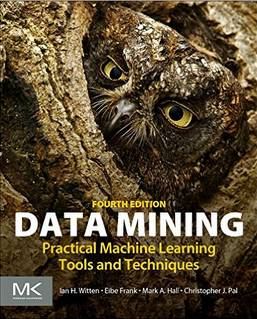# 资源|28本必读的经典机器学习/数据挖掘书籍（免费下载）Real World Machine Learning（现实世界中的机器学习）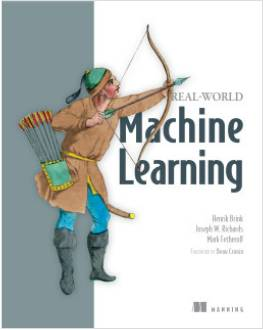An Introduction To Statistical Learning（统计学习介绍）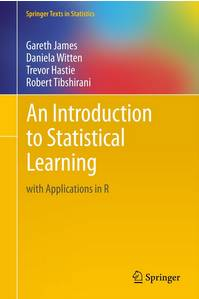An Introduction To Statistical Learning（统计学习的要素）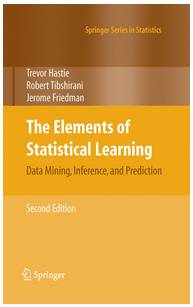Probabilistic Programming & Bayesian Methods for Hackers （概率编程与黑客的贝叶斯方法）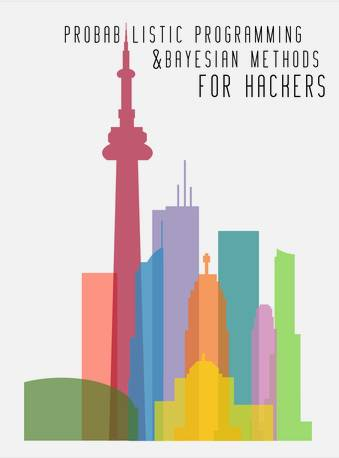Think Bayes（贝叶斯方法）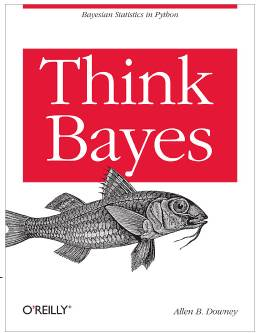Information Theory, Inference, and Learning Algorithms（信息理论，推理和学习算法）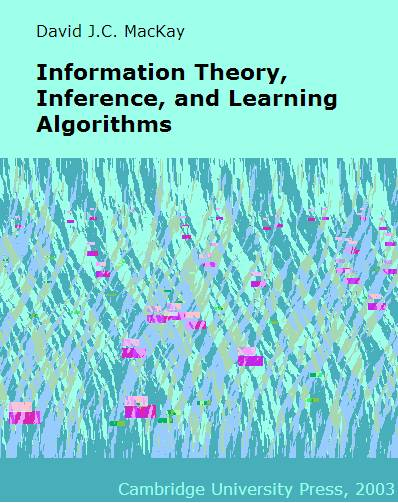Gaussian Processes for Machine Learning（机器学习的高斯过程）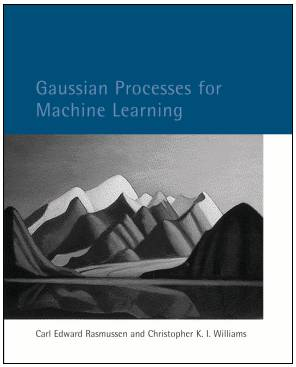Data-Intensive Text Processing with MapReduce（数据强化文本处理）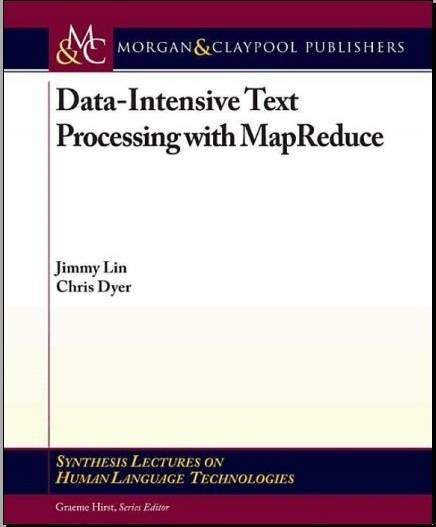Reinforcement Learning: – An Introduction（增强学习）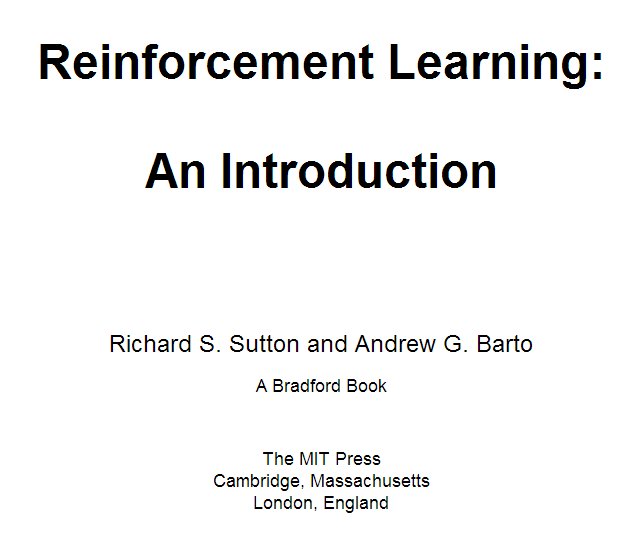Mining of Massive Datasets（数据集挖掘规模化）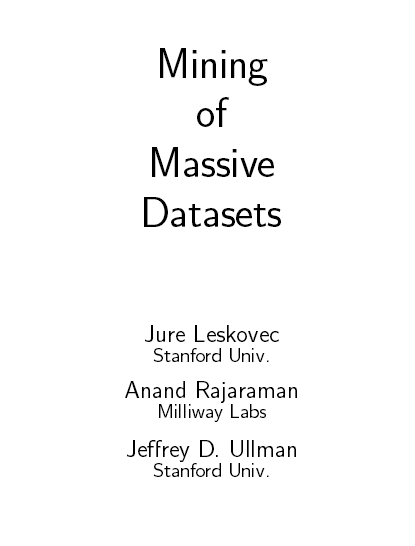A First Encounter with Machine Learning（机器学习的第一次邂逅）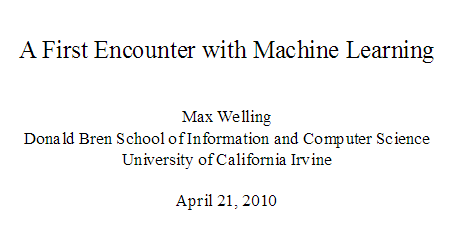Pattern Recognition and Machine Learning（模式识别和机器学习）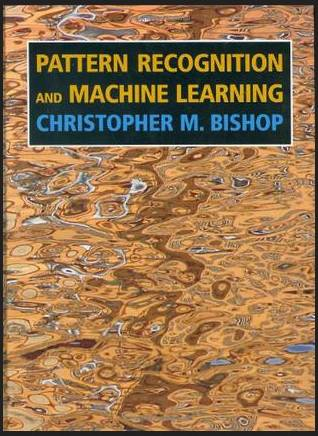Machine Learning & Bayesian Reasoning（机器学习与贝叶斯推理）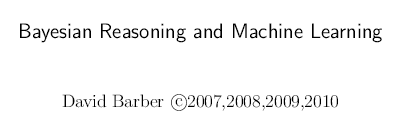Introduction to Machine Learning（机器学习简介）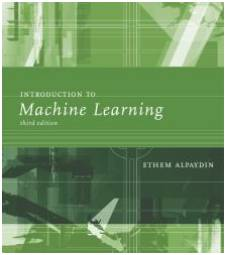A Probabilistic Theory of Pattern Recognition（模式识别的概率理论）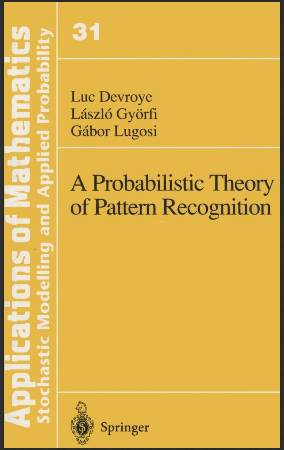ntroduction to Information Retrieval（信息检索简介）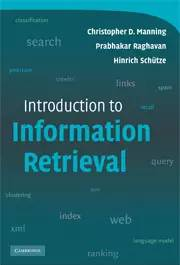Forecasting: principles and practice（预测：原则和实践）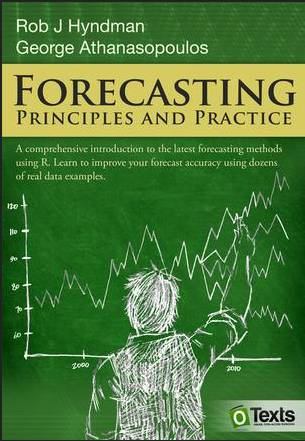Introduction to Machine Learning(机器学习简介)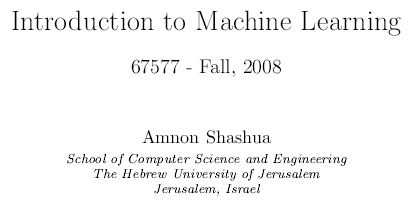Reinforcement Learning(强化学习)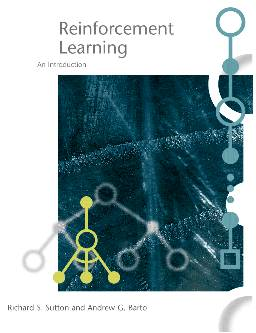Machine Learning(机器学习)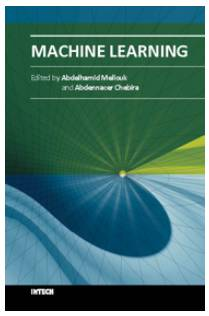A Quest for AI(AI的任务)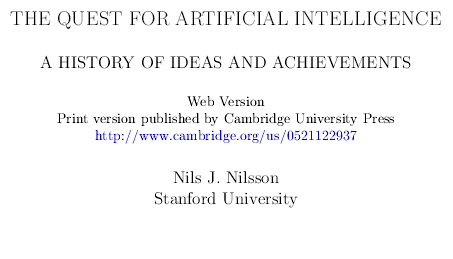Introduction to Applied Bayesian Statistics and Estimation for Social Scientists(应用贝叶斯统计学概论和社会科学家的估计)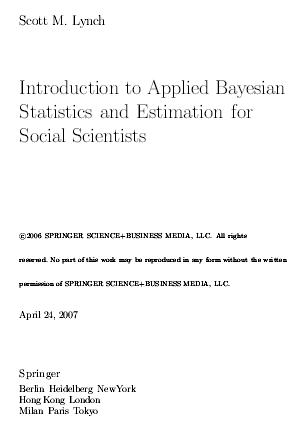Bayesian Modeling, Inference and Prediction（贝叶斯建模，推理和预测）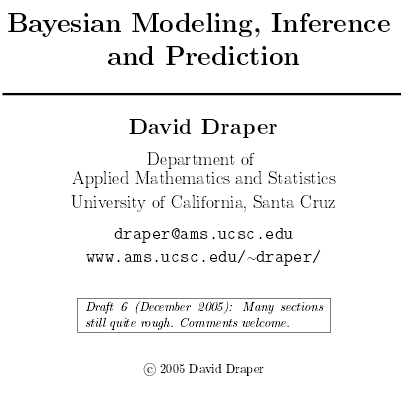A Course in Machine Learning（机器学习课程）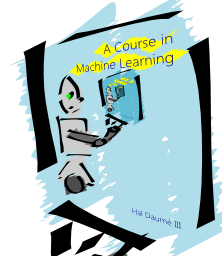Machine Learning, Neural and Statistical Classification（机器学习，神经和统计分类）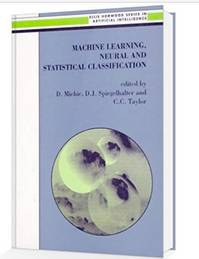Bayesian Reasoning and Machine Learning（贝叶斯推理和机器学习）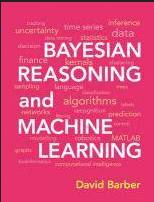R Programming for Data Science（R语言数据科学）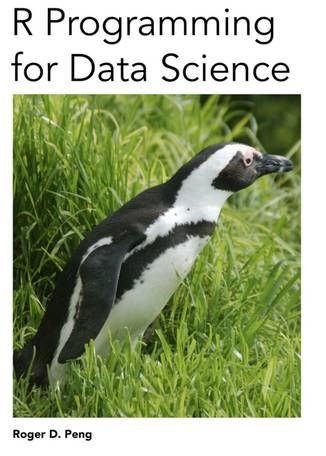Data Mining – Practical Machine Learning Tools and Techniques（数据挖掘- 实用机器学习工具和技术）